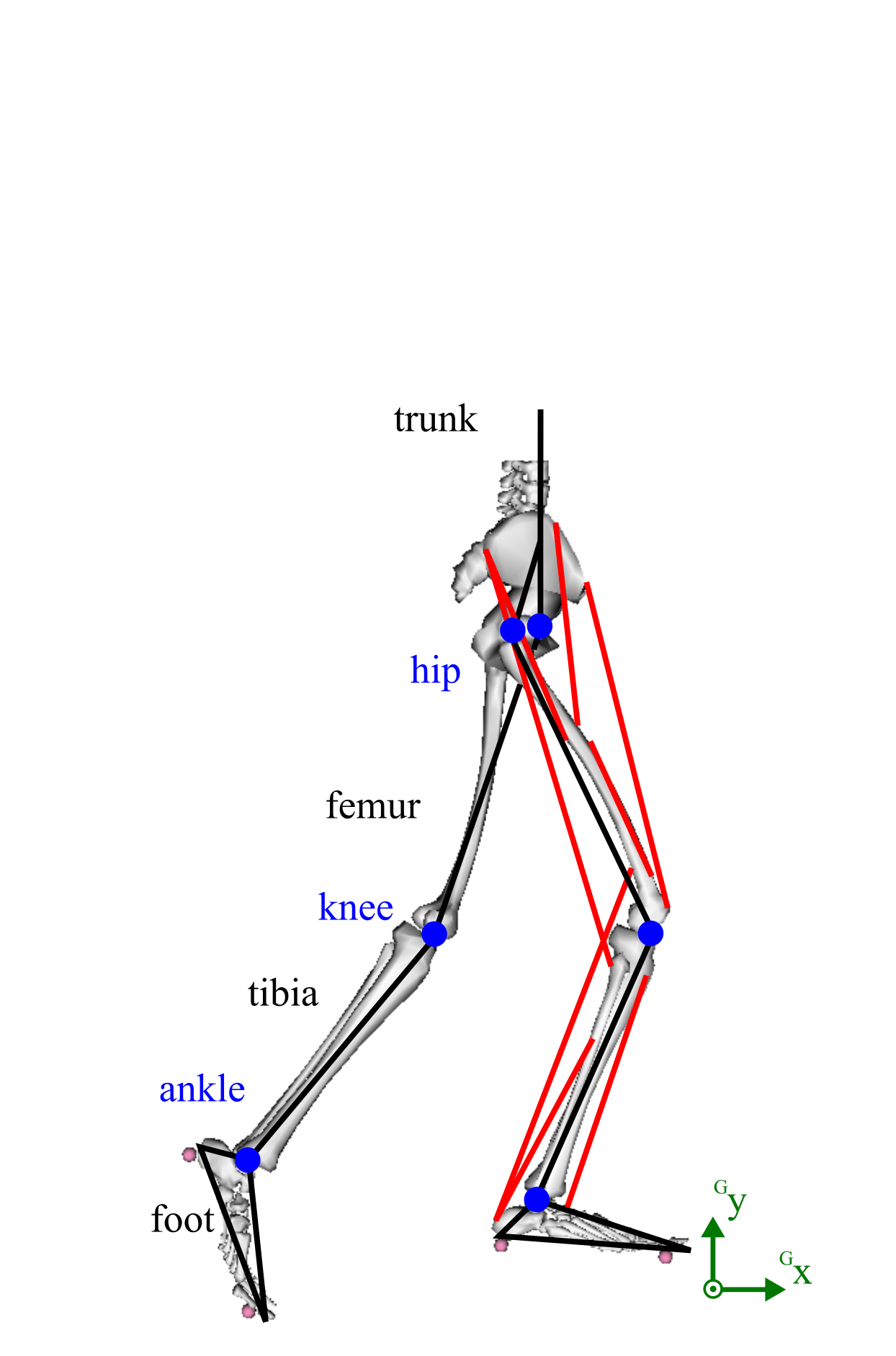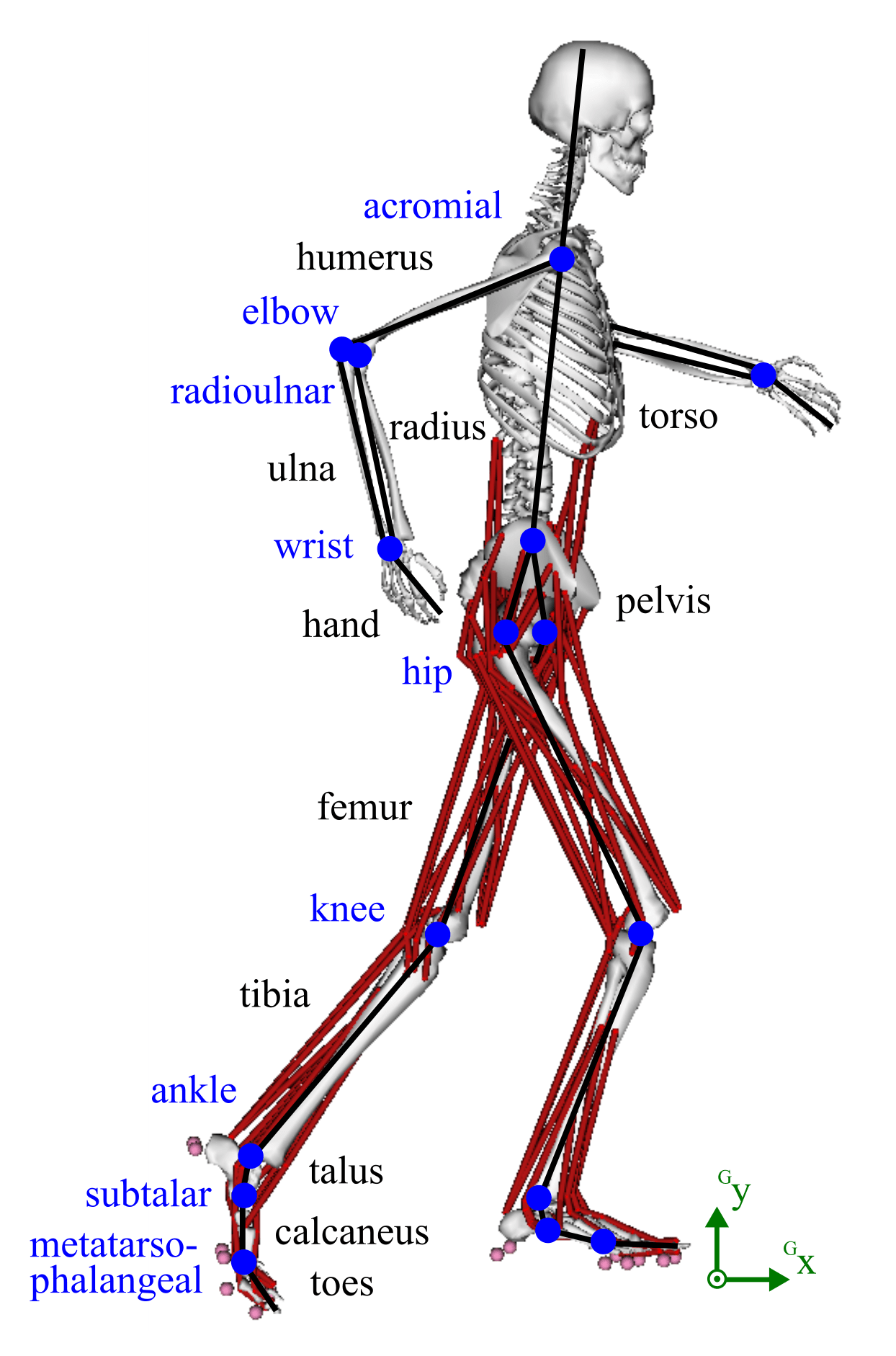# Musculoskeletal Modeling

We use musculoskeletal models to analyze and create human motion. These physical models combine multi-body dynamics, muscle activation dynamics and ground contact equations. We formulate the model dynamics implicitly to obtain efficient simulations:

$$
\mathbf{f}(\mathbf{x}(t), \mathbf{\dot{x}}(t), \mathbf{u}(t)) = \mathbf{0}
$$

The musculoskeletal models are adapted based on the research question. These are our default models in 2D and 3D: